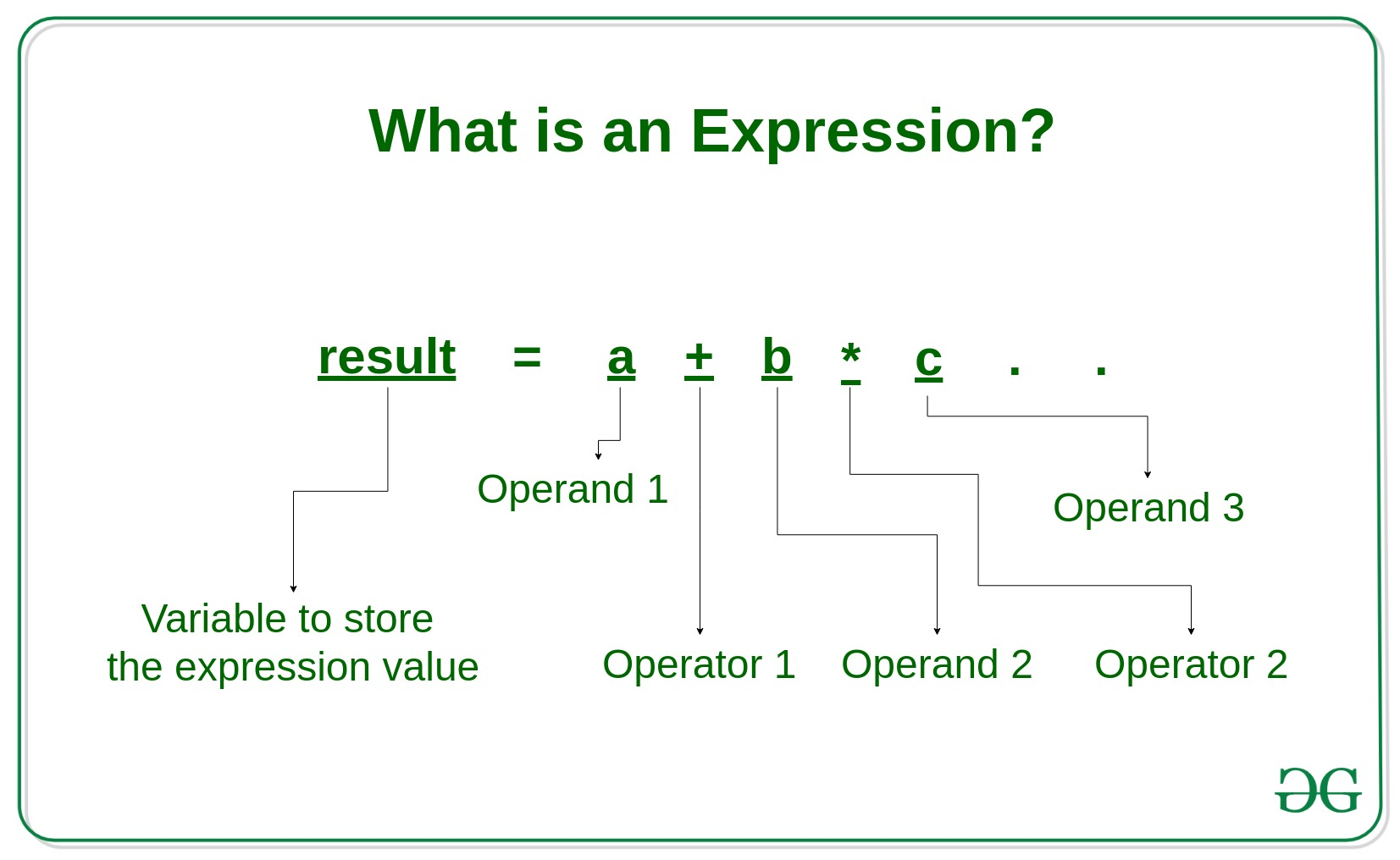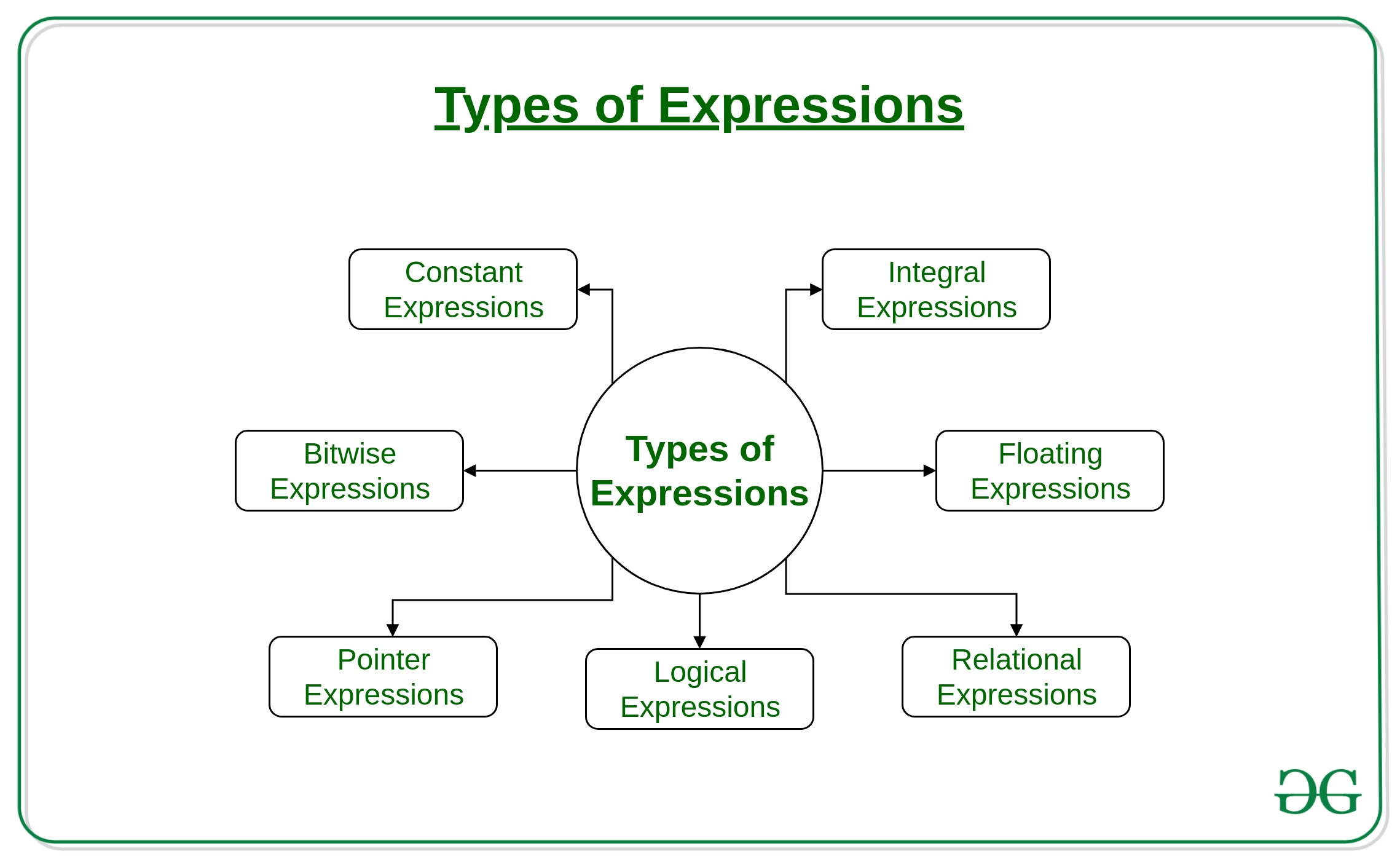# What is an Expression and What are the types of Expressions?

Expression: An expression is a combination of operators, constants and variables. An expression may consist of one or more operands, and zero or more operators to produce a value.Example:

```a+b
c
s-1/7*f
.
.
etc
```

Types of Expressions:

Expressions may be of the following types:• Constant expressions: Constant Expressions consists of only constant values. A constant value is one that doesn’t change.
Examples:

`5, 10 + 5 / 6.0, 'x’`
• Integral expressions: Integral Expressions are those which produce integer results after implementing all the automatic and explicit type conversions.
Examples:

`x, x * y, x + int( 5.0)`

where x and y are integer variables.

• Floating expressions: Float Expressions are which produce floating point results after implementing all the automatic and explicit type conversions.
Examples:

`x + y, 10.75`

where x and y are floating point variables.

• Relational expressions: Relational Expressions yield results of type bool which takes a value true or false. When arithmetic expressions are used on either side of a relational operator, they will be evaluated first and then the results compared. Relational expressions are also known as Boolean expressions.
Examples:

`x <= y, x + y > 2`
• Logical expressions: Logical Expressions combine two or more relational expressions and produces bool type results.
Examples:

` x > y && x == 10, x == 10 || y == 5 `
• Pointer expressions: Pointer Expressions produce address values.
Examples:

`&x, ptr, ptr++`

where x is a variable and ptr is a pointer.

• Bitwise expressions: Bitwise Expressions are used to manipulate data at bit level. They are basically used for testing or shifting bits.
Examples:

`x << 3`

shifts three bit position to left

`y >> 1`

shifts one bit position to right.

Shift operators are often used for multiplication and division by powers of two.

Note: An expression may also use combinations of the above expressions. Such expressions are known as compound expressions.

Attention reader! Don’t stop learning now. Get hold of all the important DSA concepts with the DSA Self Paced Course at a student-friendly price and become industry ready.

My Personal Notes arrow_drop_upCheck out this Author's contributed articles.

If you like GeeksforGeeks and would like to contribute, you can also write an article using contribute.geeksforgeeks.org or mail your article to contribute@geeksforgeeks.org. See your article appearing on the GeeksforGeeks main page and help other Geeks.

Please Improve this article if you find anything incorrect by clicking on the "Improve Article" button below.